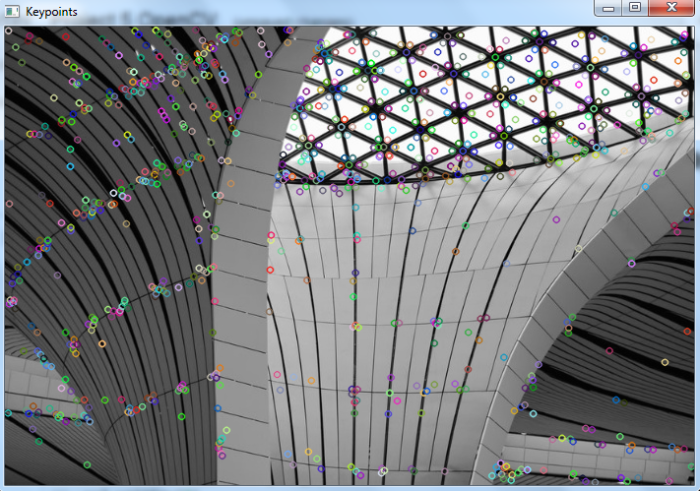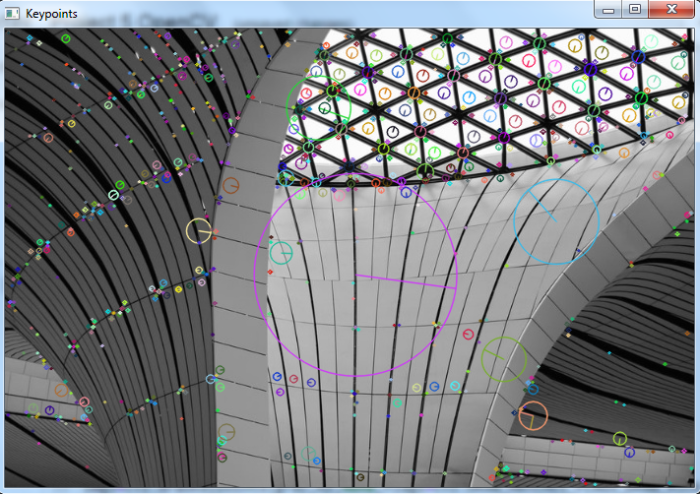# OpenCV Python – How to detect and draw keypoints in an image using SIFT?

SIFT (Scale-Invariant Feature Transform ) is scale invariant feature descriptor. It detects keypoints in the image and computes its descriptors. We first create a SIFT object with cv2.SIFT_create(). Then detect the keypoints using sift.detect() where sift is the created SIFT object. To draw keypoints, we use cv2.drawKeypoints().

## Steps

To detect and draw keypoints in the input image using SIFT algorithm, you could follow the steps given below

• Import the required libraries OpenCVand NumPy. Make sure you have already installed them.

• Read the input image using cv2.imread() method. Specify the full path of the image. Convert the input image to grayscale image using cv2.cvtColor() method.

• Initiate SIFT object with default values using sift=cv2.SIFT_create().

• Detect the keypoints in the grayscale image. Use sift.detect(). It returns keypoints kp.

• Draw the detected keypoint kp on the image cv2.drawKeypoints() function. To draw rich keypoints you can pass flags=cv2.DRAW_MATCHES_FLAGS_DRAW_RICH_KEYPOINTS as a parameter.

• Display the image with drawn keypoints on it

Let's see the examples to detect and draw keypoints in the input image using the SIFT algorithm.

## Input Image

We will use the following image as the input file in the examples below.## Example

In this program, we detect and draw keypoints in the input image using the SIFT algorithm.

# import required libraries
import cv2

# convert the image to grayscale
gray = cv2.cvtColor(img, cv2.COLOR_BGR2GRAY)

# Initiate SIFT object with default values
sift = cv2.SIFT_create()

# find the keypoints on image (grayscale)
kp = sift.detect(gray,None)

# draw keypoints in image
img2 = cv2.drawKeypoints(gray, kp, None, flags=0)

# display the image with keypoints drawn on it
cv2.imshow("Keypoints", img2)
cv2.waitKey(0)
cv2.destroyAllWindows()


On execution of the above code, it will open the following output window, showing the image with drawn keypoints on it.Notice that the keypoints are drawn with different colors. You can pass color (ie. for red (0,0,255)) as a parameter to the drawKeypoints() function to draw keypoints with a single color.

## Example

In this example, we will see how to detect and draw keypoints in the input image using SIFT algorithm.

We pass flags=cv2.DRAW_MATCHES_FLAGS_DRAW_RICH_KEYPOINTS to cv2.drawKeypoints() as a parameter.

# import required libraries
import cv2

# convert the image to grayscale
gray = cv2.cvtColor(img, cv2.COLOR_BGR2GRAY)

# Initiate SIFT object with default values
sift = cv2.SIFT_create()

# find the keypoints on image (grayscale)
kp = sift.detect(gray,None)

# draw keypoints in image
img2=cv2.drawKeypoints(gray,kp,None,flags=cv2.DRAW_MATCHES_FLAG
S_DRAW_RICH_KEYPOINTS)

# display the image with keypoints drawn on it
cv2.imshow("Keypoints", img2)
cv2.waitKey(0)
cv2.destroyAllWindows()


## Output

On execution of the above code, it will open the following output window, showing the image with drawn keypoints on it.Notice that the keypoints are drawn with different sizes and even with orientation.